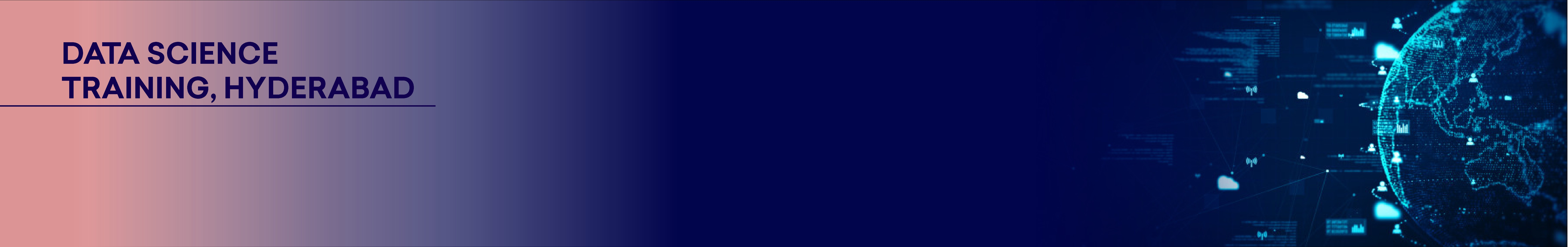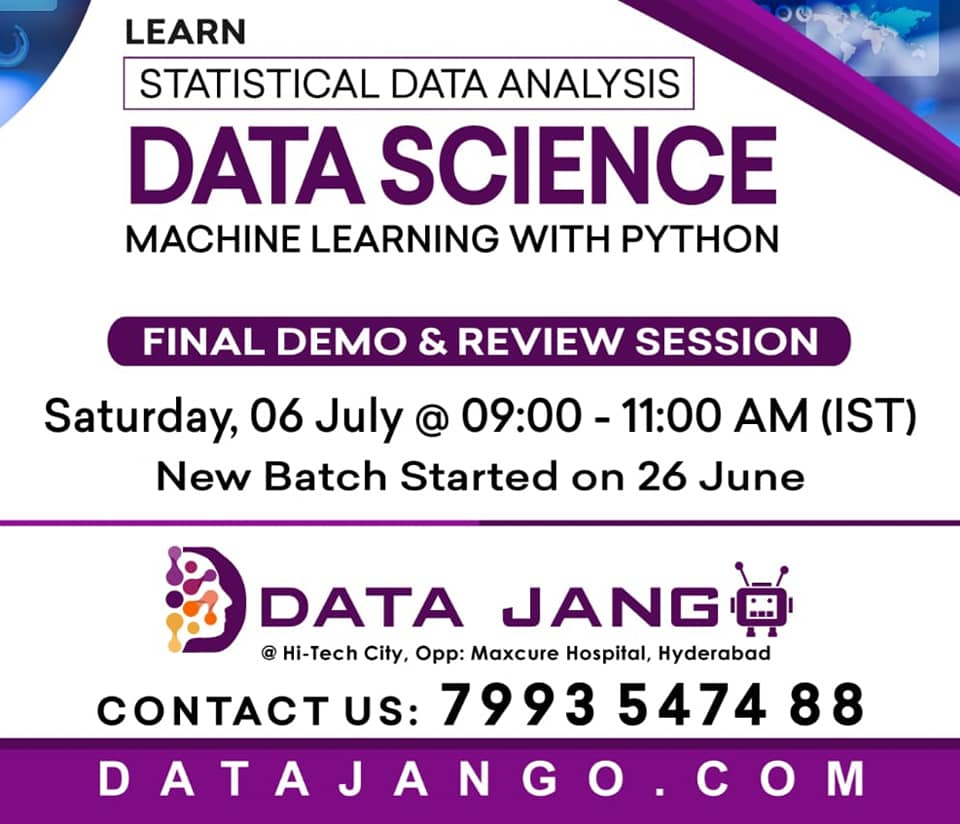# Data Science Training in Hyderabad

Data Jango providing real time training in the fields of Data Science like Machine Learning which covers Supervised Learning, Unsupervised Learning and Reinforcement Learning, Deep Learning which covers Neural Networking Concepts like ANN, CNN and RNN using Keras, NLP, NLU which will help in building ai based applications.

Mode of Training: Online and Class Room(Hyderabad).

Duration: 3 Months

Push yourself, Because no one else is going to do IT for You — Success Quote

The course we will cover all necessary concepts to make a successful Data Scientist. The concepts we cover are Descriptive Statistics, Inferential Statistics, Basic Python, Pandas, NumPy, SciPy, Statistical Data Analysis, StatsModels, Scikit-Learn, Mathematics behind Machine Learning Algorithms (Gradient Descent, SVM, Kernal SVM, etc.), error analysis and most of the accuracy measures, techniques of fine-tuning a model.

### Details of Data Science Training in Hyderabad:

Data Jango Training institute located in Madhapur, Hyderabad designed Data Science course in two phases, First one focuses on Machine Learning, Second one Focuses on Deep Learning.

 Course Location Mode of Class Duration Data Science Hyderabad Class-Room/Online 3 Months

745 Ratings

### Data Science Course HighlightsTrainer with 10+ years of experience
Certified Trainer
Real-Time Project Examples
Exercises after every Topic
Trainer support after completion of the course
Placement Assistance
Trainers with Industry Experience and IIIT Hyderabad
All Concepts and ML/DL Algorithms with Code Examples

### Descriptive Statistics

• Central Tendency (mean, median and mode)
• Interquartile Range
• Variance
• Standard Deviation
• Z-Score/T-Score
• Co-variance
• Correlation

### Data Distributions

• Binomial Distribution
• Introduction to Probability
• Normal Distribution

### Overview of Data Visualization

• Bar Chart
• Histogram
• Box whisker plot
• Dot-plot
• Line plot
• Scatter Plot

### Introduction to Python

• How to install python (anaconda)
• How to work with Jupyter Notebook
• How to work with Spyder IDE
• Compound data types
o Strings, Lists, Tuples, Sets, Dictionaries
• Control Flows
• Keywords (continue, break, pass)
• Functions (Formal/Positional/Keyword arguments)
• Predefined functions (range, len, enumerate, zip)

### Introduction to NumPy

• One-dimensional Array
• Two-dimensional Array
• Predefined functions (arrange, reshape, zeros, ones, empty, eye, linespace)
• Basic Matrix operations
o Slicing, indexing, Looping, Shape Manipulation, Stacking

• Series
• DataFrame
• df.GroupBy
• df.crosstab
• df.apply
• df.map
• df.mapapply

### Inferential Statistics

• Central Limit Theorem
• Confidence Interval and z-distribution table
• Statistical Significance
• Hypothesis testing
• P-value
• One-tailed and Two-tailed Tests
• Chi-Square Goodness of Fit Test
• F- Statistic (ANOVA)
• Skewness, Kurtosis

### Exploratory Data Analysis

• Train/Test split – Data snooping bias
• Statistical Data Analysis
• Fixing missing values
• Finding outliers
• Data quality check
• Feature transformation
• Data Visualization (Matplotlib, Seaboarn)
o Categorical to Categorical
o Categorical to Quantitative
o Quantitative to Quantitative
• Bi-Variate data analysis (Hypothesis Testing)
o Categorical and Quantitative (ANOVA)
o Categorical to Categorical (Chi-Square)
o Quantitative to Categorical (Chi-Square)
o Quantitative to Quantitative (Correlation)

### Intro to Regression (Supervised Learning)

• What is regression?
• Simple linear regression
• Linear Regression – a statistics perspective (statsmodels – OLS)
• Evaluation metrics (R-Squre, Adj R-Squre, MSE, RMSE)

### Regression Analysis (ML – statsmodels)

• Mean centralization and its use in multiple linear regression
• Multiple linear regression
• P – Value based feature selection methods (Backward, Forward and Mixed)
• Linear regression assumptions (linear relations – fitted vs residuals plot, homoscedasticity, normal distribution of error term, serial correlation, multicollinearity)
• Q-Q Plot, Shapiro Wilk test – different ways to check normality of data.
• Data transformation techniques.

### Encoding & Code Modularization

• Label Encoding
• One-Hot (dummy variable) encoding
• Dummy variable trap
• Scikit-Learn → Custom Transformers
• Scikit-Learn → Pipeline

### Multiple Linear regression (scikit-learn)

• Normal Equation (Linear Algebraic way of solving linear equation)
• Gradient Descent (Calculus way of solving linear equation)
• Multiple Linear Regression (SGD Regressor)
• Feature Scaling ( Min-Max vs Mean Normalization)
• Feature Transformation
• Polynomial Regression

### Model Evaluation, Model Selection, Polynomial Regression, Regularization.

• Train/Validation/Test split
• K-Fold Cross Validation
• The Problem of Over-fitting (Bias-Variance tread-off)
• Learning Curve
• Regularization (Ridge, Lasso and Elastic-Net)
• Feature selection
• Hyper Parameter Tuning (GridSearchCV, RandomizedSearchCV)

### Model Deployment

• Pickle (pkl file)
• Model load from pkl file and prediction

### Classification (Supervised Learning)

• Logistic Regression Algorithm (SGD Classifier)
• Accuracy measurements – handling imbalanced dataset
o Accuracy score
o Confusion matrix
o Precision
o Recall
o Precision – Recall tread off curve
o ROC curve
o AUC score
• Multi-class Classification
o One-vs-One
o One-vs-All
o Softmax regression classifier
• Multi-label Classification
• Multi- output Classification

### Support Vector Machine

• SVM Classifier (Soft/Hard – Margin)
• Linear SVM
• Non-Linear SVM
• Kernel Trick (mathematics behind kernel trick)
• Kernel SVM
• SVM Regression

### Clustering (Unsupervised Learning)

• K-means
• Hierarchical
• How to use unsupervised outcome as support to solve supervised problem.

### Dimensionality Reduction (Unsupervised)

• PCA
• Math behind PCA – Eigen vectors, eigen values, covariance matrix.
• Choosing Right Number of Dimensions or Principal Components
• Incremental PCA
• Kernel PCA

### Tree Based Algorithms

• Regression Trees vs Classification Trees
• Entropy
• Gini Index
• Information Gain
• Tree pruning

### Ensemble models

• Voting Classifiers (Heterogeneous Ensemble Models)
• Homogeneous Ensemble Models
o Random Forest
o Bagging
o Pasting

### Naive Bayes

• Bayes Theorem
• Naive Bayes Algorithm
• Introduction to Text Analytics
• Tokenization
• Text Normalization, stemming, lemmatization
• Bag of words mode

### Anomaly Detection

• Anomaly vs Classification
• Credit Card Fraud detection – Anomaly Detection Algorithm
• Assumptions of normality

### Introduction to Hadoop & PySpark

• Overview of YARN architecture
• Map-Reduce example
• Overview of Spark Context (–master YARN)
• Resilient Distributed Datasets (RDDs)
• RDD Operations (Transformations, Actions)
• Spark DataFrames
• Spark ML model with Pipeline
• Classification model, MulticlassMetrics

### Introduction to Neural Networks

• Perceptron, Sigmoid Neuron
• Neural Network model representation
• How it works
• Forward-Propagation
• Back-Propagation

## Testimonials By Data Science Course Learners

### Do one need to have some computer programming knowledge ?

• It is better to have minimum programming knowledge.
• We are anyway providing necessary programming skills to execute Data Science projects.

### Explain course design and duration?

• The course will make you a solution provided for the real-time Data Science problems.
• We start with Statistics, Basics of Python, data analysis with Pandas, NumPy, after this we will get deeper into Machine Learning, Error analysis and Fine tuning models.
• The course duration is of three months, every day one hour. Monday to Friday.

### Can we attend class online ?

• Yes, we broadcast live class over the internet. One could join the class either in classroom or online.
• We are also providing recorded videos for further reference.

### Who can become a Data Scientist ?

• Business Analysts and Data Analysts.
• Database professionals, Developers, Leads, Managers from Information Technology industry.
• Fresh graduates who wish to make a career in Data Science, Machine Learning, Statistical Data Analysis and Artificial Intelligence.

### What are the prerequisites to join Data Science course ?

• We will cover all that is needed to make you a Data Scientist (Statistics, Mathematics, Machine Learning, Python, etc..).
• Only requirements are, should have studied mathematics at 10 + 2 (Intermediate) level. Good intuition and logic.

### How soon I can get a job as per current market situation?

• We would say, with in one month of finishing the course. This depends on below conditions.
• The course duration will take three months, we impart all concepts with utmost clarity and depth. The course material covers answers for all most all interview questions. This will build lot of confidence to face interview. It requires dedication and lot of practice of the concepts we taught. Practice makes prefect.
DataJango - Chatbot